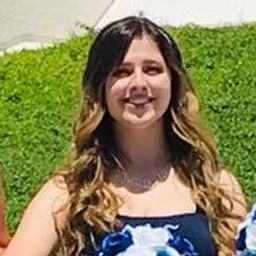## Educators

PP
RD+ 1 more educators

### Problem 1

Solve the differential equation.

$y'' - y' - 6y = 0$

PP
Pravakar P.

### Problem 2

Solve the differential equation.

$y'' - 6y' + 9y = 0$

PP
Pravakar P.

### Problem 3

Solve the differential equation.

$y'' + 2y = 0$

PP
Pravakar P.

### Problem 4

Solve the differential equation.

$y'' + y' - 12y = 0$

PP
Pravakar P.

### Problem 5

Solve the differential equation.

$4y'' + 4y' + y = 0$

PP
Pravakar P.

### Problem 6

Solve the differential equation.

$9y'' + 4y = 0$

PP
Pravakar P.

### Problem 7

Solve the differential equation.

$3y'' = 4y'$

PP
Pravakar P.

### Problem 8

Solve the differential equation.

$y = y''$

PP
Pravakar P.

### Problem 9

Solve the differential equation.

$y'' - 4y' + 13y = 0$

PP
Pravakar P.

### Problem 10

Solve the differential equation.

$3y'' + 4y' - 3y = 0$

PP
Pravakar P.

### Problem 11

Solve the differential equation.

$2 \dfrac{d^2y}{dt^2} + 2 \dfrac{dy}{dt} - y = 0$

PP
Pravakar P.

### Problem 12

Solve the differential equation.

$\dfrac{d^2R}{dt^2} + 6 \dfrac{dR}{dt} + 34R = 0$

PP
Pravakar P.

### Problem 13

Solve the differential equation.

$3 \dfrac{d^2V}{dt^2} + 4 \dfrac{dV}{dt} + 3V = 0$

PP
Pravakar P.

### Problem 14

Graph the two basic solutions along with several other solutions of the differential equation. What features do the solutions have in common?

$4 \dfrac{d^2y}{dx^2} - 4 \dfrac{dy}{dx} + y = 0$

Check back soon!

### Problem 15

Graph the two basic solutions along with several other solutions of the differential equation. What features do the solutions have in common?

$\dfrac{d^2y}{dx^2} + 2 \dfrac{dy}{dx} + 2y = 0$

Check back soon!

### Problem 16

Graph the two basic solutions along with several other solutions of the differential equation. What features do the solutions have in common?

$2 \dfrac{d^2y}{dx^2} + \dfrac{dy}{dx} - y = 0$

RD
Richard D.

### Problem 17

Solve the initial-value problem.

$y" + 3y = 0$, $y(0) = 1$, $y'(0) = 3$

PP
Pravakar P.

### Problem 18

Solve the initial-value problem.

$y" - 2y' - 3y = 0$, $y(0) = 2$, $y'(0) = 2$

PP
Pravakar P.

### Problem 19

Solve the initial-value problem.

$9y" + 12y' + 4y = 0$, $y(0) = 1$, $y'(0) = 0$

PP
Pravakar P.

### Problem 20

Solve the initial-value problem.

$3y" - 2y' - y = 0$, $y(0) = 0$, $y'(0) = -4$Dharmendra J.

### Problem 21

Solve the initial-value problem.

$y" - 6y' + 10y = 0$, $y(0) = 2$, $y'(0) = 3$Dharmendra J.

### Problem 22

Solve the initial-value problem.

$4y" - 20y' + 25y = 0$, $y(0) = 2$, $y'(0) = -3$Dharmendra J.

### Problem 23

Solve the initial-value problem.

$y" - y' - 12y = 0$, $y(1) = 0$, $y'(1) = 1$Dharmendra J.

### Problem 24

Solve the initial-value problem.

$4y" + 4y' + 3y = 0$, $y(0) = 0$, $y'(0) = 1$Dharmendra J.

### Problem 25

Solve the boundary-value problem, if possible.

$y" + 16y = 0$, $y(0) = -3$, $y(\pi/8) = 2$Dharmendra J.

### Problem 26

Solve the boundary-value problem, if possible.

$y'' + 6y' = 0$, $y(0) = 1$, $y(1) = 0$Dharmendra J.

### Problem 27

Solve the boundary-value problem, if possible.

$y'' + 4y' + 4y = 0$, $y(0) = 2$, $y(1) = 0$Dharmendra J.

### Problem 28

Solve the boundary-value problem, if possible.

$y'' - 8y' + 17y = 0$, $y(0) = 3$, $y(\pi) = 2$Dharmendra J.

### Problem 29

Solve the boundary-value problem, if possible.

$y'' = y'$, $y(0) = 1$, $y(1) = 2$Dharmendra J.

### Problem 30

Solve the boundary-value problem, if possible.

$4y'' - 4y' + y = 0$, $y(0) = 4$, $y(2) = 0$### Problem 31

Solve the boundary-value problem, if possible.

$y" + 4y' + 20y = 0$, $y(0) = 1$, $y(\pi) = 2$Jeffrey U.

### Problem 32

Solve the boundary-value problem, if possible.

$y" + 4y' + 20y = 0$, $y(0) = 1$, $y(\pi) = e^{-2\pi}$Dharmendra J.

### Problem 33

Let $L$ be a nonzero real number.

(a) Show that the boundary-value problem $y'' + \lambda y = 0$, $y(0) = 0$, $y(L) = 0$ has only the trivial solution $y = 0$ for the cases $\lambda = 0$ and $\lambda < 0$.

(b) For the case $\lambda > 0$, find the values of $\lambda$ for which this problem has a nontrivial solution and give the corresponding solution.Dharmendra J.

### Problem 34

If $a$, $b$, and $c$ are all positive constants and $y(x)$ is a solution of the differential equation $ay'' + by' + cy = 0$, show that $\lim_{x \to \infty} y(x) = 0$.

Check back soon!

### Problem 35

Consider the boundary-value problem $y''- 2y' + 2y = 0$, $y(a) = c$, $y(b) = d$.

(a) If this problem has a unique solution, how are $a$ and $b$ related?

(b) If this problem has no solution, how are $a$, $b$, $c$, and $d$ related?

(c) If this problem has infinitely many solutions, how are $a$, $b$, $c$, and $d$ related?Samantha L.